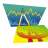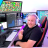# Code backtesting script based on Linear regression and moving average?

A

##### Member
Hey!

First of all not a coding wizard (you probably guessed by the title) but I have an interesting strategy to share and request help for converting this into a strategy for backtesting. Idk the source for this but I made some tweaks and here are the rules:

Longs:
Entry:
1. The 21 Period Linear regression curve > 100 Period Linear regression curve > 100 Period Simple Moving Average
2. The Aroon Up plot > Aroon Down plot
3. The slope of the 100 SMA > 0

Exit:
1. When any of those conditions are invalidated
-- or at an area (this one is on you)

Shorts are the exact opposite.

I have been using it mainly on the 1 Minute chart on futures (mainly /ES) and have got great results so far

Here is my chart w a custom slope indicator for the SMA: https://tos.mx/AmJUiJ1

Here are some example trades (these are on /ES):

Longs:
Trade 1: Entry: 3118, Exit: 3121 = 3 Points up
Trade 2: 3124.75 - 3124.25 = -0.5 Points down
Trade 3: 3127.5 - 3127.5 = Break evenShorts:
Trade 1: Entry: 3052.5, Exit: 3011.25 = 41.25 Points up (*granted, not every trade will be like this)I'll try my best to answer questions and open to discussion regarding the strat.

Thanks guys!Last edited:
M

#### mashume

##### Active member
VIP
Perhaps something like this:

Code:
``````# 1. The 21 Period Linear regression curve > 100 Period Linear regression curve > 100 Period Simple Moving Average
# 2. The Aroon Up plot > Aroon Down plot
# 3. The slope of the 100 SMA > 0

def cond_1 = linearRegCurve(length=21) > linearRegCurve(100);
def cond_2 = AroonIndicator().up > aroonIndicator().down;
def cond_3 = SimpleMovingAvg(length=100) > SimpleMovingAvg(length=100);

addOrder(OrderType.SELL_TO_CLOSE, !cond_1 or !cond_2 or !cond_3);``````
Not sure what the results will look like, and this uses BUY_TO_OPEN rather than BUY_AUTO. Change as you see fit.

-mashume

Last edited:
•BenTen
A

##### Member
First of all, thanks for your time and effort for this. This is taking some trades that don't fit the rules, for one; when defining cond_1 no clause for the two averages also being above the 100 SMA. It is also taking trades that I don't understand and appear to have no catalyst.

I altered the code for def cond_1 but now no orders are showing up at allhere it is:

Code:
``````# 1. The 21 Period Linear regression curve > 100 Period Linear regression curve > 100 Period Simple Moving Average
# 2. The Aroon Up plot > Aroon Down plot
# 3. The slope of the 100 SMA > 0

def cond_1 = linearRegCurve(length=21) > linearRegCurve(100) > SimpleMovingAvg(length=100);
def cond_2 = AroonIndicator().up > aroonIndicator().down;
def cond_3 = SimpleMovingAvg(length=100) > SimpleMovingAvg(length=100);

addOrder(OrderType.SELL_TO_CLOSE, !cond_1 or !cond_2 or !cond_3);``````

also tried splitting up the greater than conditions:

Code:
``````# 1. The 21 Period Linear regression curve > 100 Period Linear regression curve > 100 Period Simple Moving Average
# 2. The Aroon Up plot > Aroon Down plot
# 3. The slope of the 100 SMA > 0

def cond_1 = linearRegCurve(length=21) > linearRegCurve(100);
def cond_2 = linearRegCurve(length=21) > SimpleMovingAvg(length=100);
def cond_3 = linearRegCurve(length=100) > SimpleMovingAvg(length=100);
def cond_4 = AroonIndicator().up > aroonIndicator().down;
def cond_5 = SimpleMovingAvg(length=100) > SimpleMovingAvg(length=100);

addOrder(OrderType.SELL_TO_CLOSE, !cond_1 or !cond_2 or !cond_3 or !cond_4 or !cond_5);``````
still to no avail...

here is an example of the trades (they don't abide by the rules):the first long got triggered for no reason, it already should have been in a long. The next trades shouldn't even be happening.

Please helpLast edited:
A

##### Member
nvm, I learnt a bit of coding and coded it myself. The results are fantastic for smaller time frame charts!

•Zillion and mashume
M

#### mashume

##### Active member
VIP
•Highlighting Code with Ruby Syntax Questions 1If then {multiple lines of code} ? Questions 2